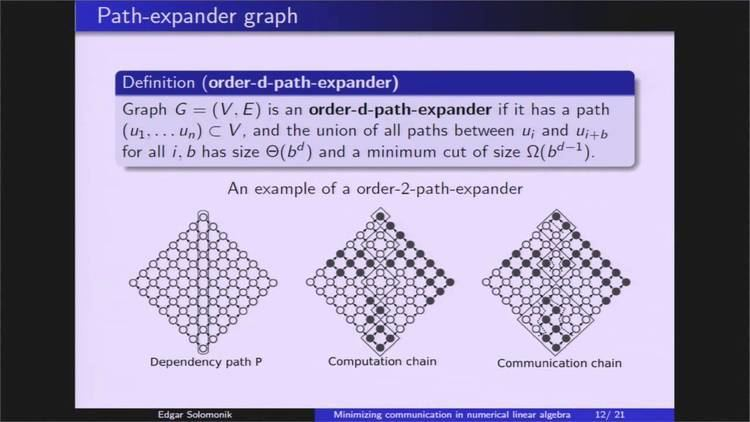# Numerical linear algebra

Updated on
Covid-19Numerical linear algebra is the study of algorithms for performing linear algebra computations, most notably matrix operations, on computers. It is often a fundamental part of engineering and computational science problems, such as image and signal processing, telecommunication, computational finance, materials science simulations, structural biology, data mining, bioinformatics, fluid dynamics, and many other areas. Such software relies heavily on the development, analysis, and implementation of state-of-the-art algorithms for solving various numerical linear algebra problems, in large part because of the role of matrices in finite difference and finite element methods.

## Contents

Common problems in numerical linear algebra include computing the following: LU decomposition, QR decomposition, singular value decomposition, eigenvalues.

## References

Similar Topics
Last Night (1964 film)
Federico Bongioanni
Khaled Mouzanar
Topics